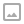## PowerShell - Print All Odd/Even Lines

·   ·  ☕ 1 min read

As a little brainstormer, suppose you have a variable with the following test content:

 1 2 3 4 5 6 7 8  $str = @" Line 1 Line 2 Line 3 Line 4 Line 5 Line 6 "@  Here is how you can print all odd lines from it:  1  $f=0; $str.Split("n") | % { if($f = !$f) {$_ } } 

Or, if you want all even lines, change the initial $f value to 1:  1  $f=1; $str.Split("n") | % { if($f = !$f) {$_ } }WRITTEN BY
Victor Zakharov
Web Developer (Angular/.NET)